## Phase Diagrams of Inhomogeneous Superconductors

#### Chuck Agosta - Clark University

Strong evidence of inhomogeneous superconductivity has been found in a number of crystalline organic superconductors. Weak evidence has been found in heavy fermion and pnictide superconductors. Most of the evidence has been based on phase diagrams built from various probes either directly or indirectly of the superconducting order parameter, in this case the Cooper pairs. Other possibly more compelling evidence comes from specific heat or NMR measurements. Below are examples of proposed phase diagrams.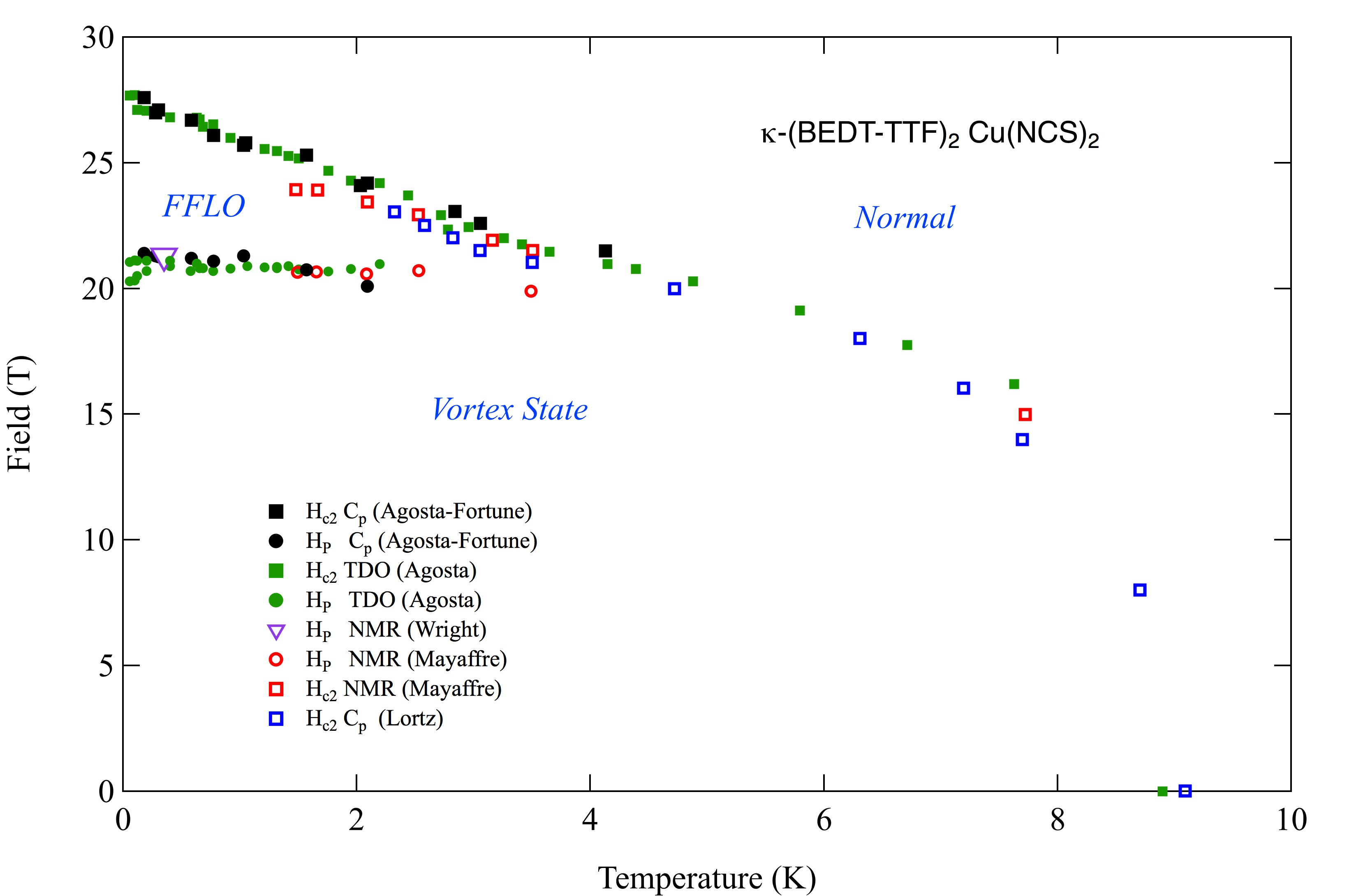$\kappa$-(BEDT-TTF)$_2$Cu(NCS)$_2$ is the material with the most data, and is the most robust candidate for inhomogeneous superconductivity. This diagram shows data from rf penetration1, specific heat2,3,and NMR4,5 measurements. The specific heat measurements have determined the order of the phase transitions and established that the entropy increases moving from the vortex state to the FFLO state. 2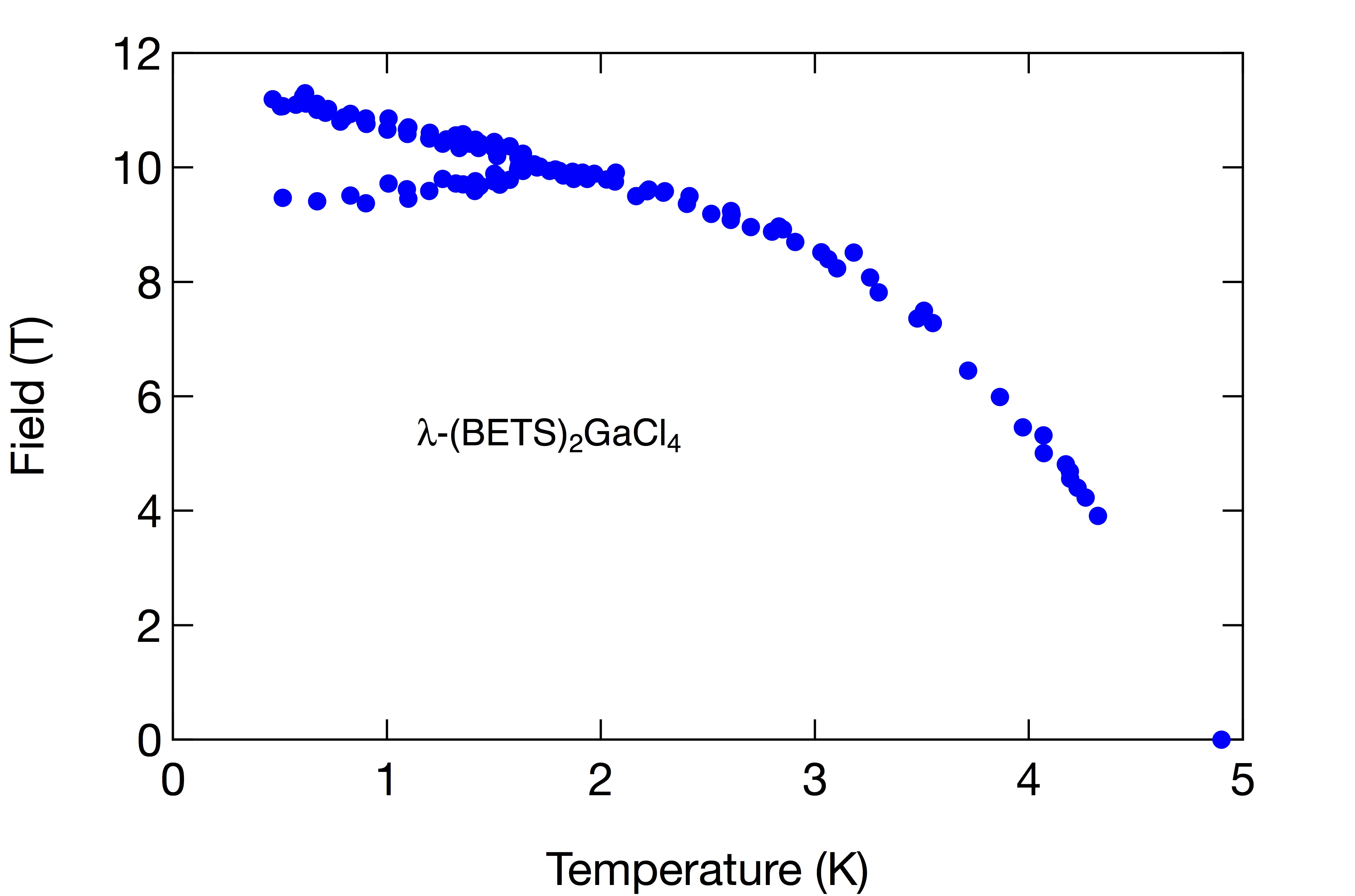$\lambda$-(BETS)$_2$GaCl$_4$ is a good sample to study because its vortex-FFLO phase line is at a reasonable magnetic field range. The high point density is due to the high throughput of our pulsed magnetic field apparatus6. This phase diagram contains 109 field sweeps. The high point density increases the confidence level of the phase lines.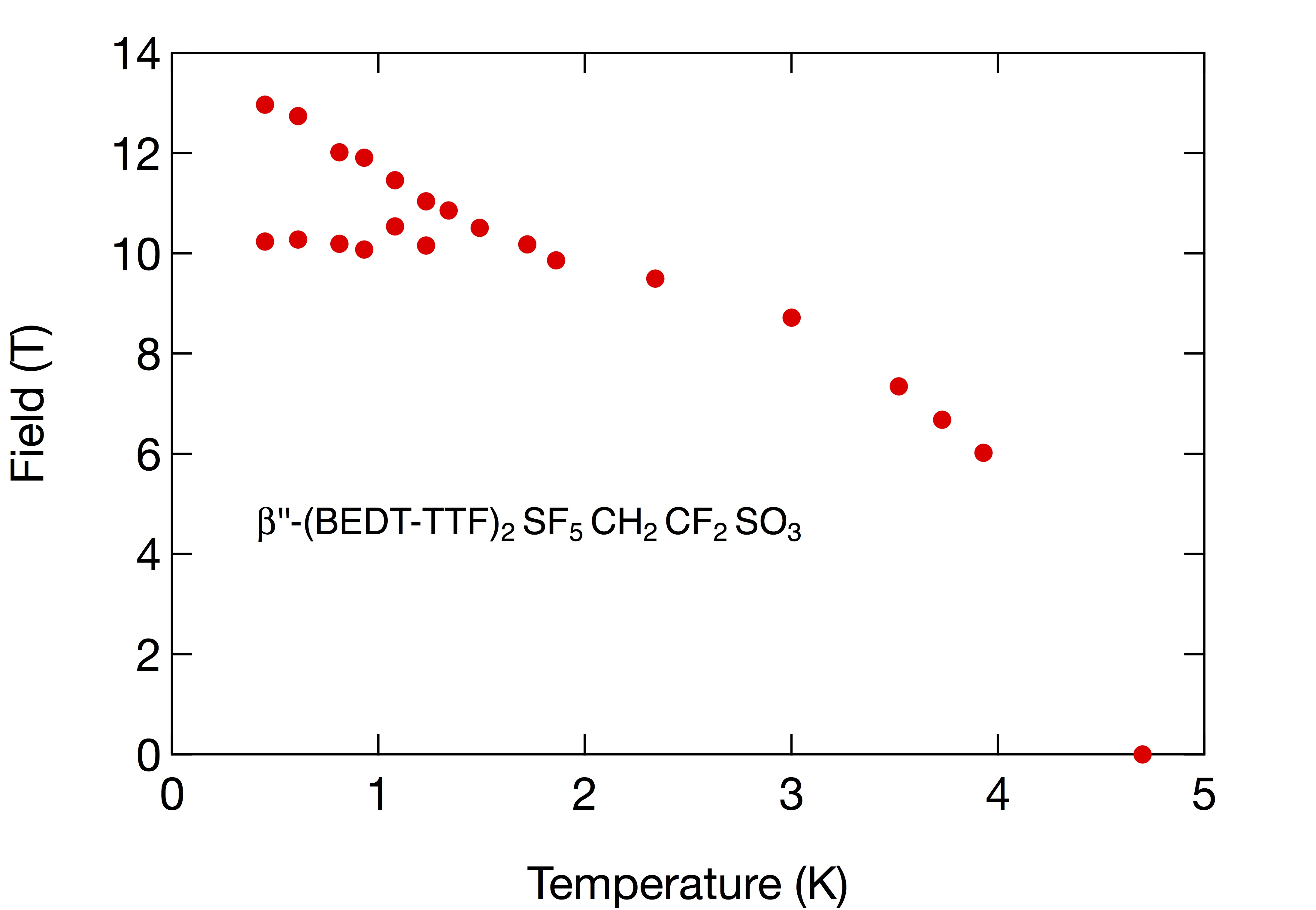$\beta$"-(BEDT-TTF)$_2$SF$_5$CH$_2$CF$_2$SO$_3$ was one of the first materials where the vortex state FFLO state phase line was found7. There is also NMR data on this sample. For reasons that we do not understand the anomaly in rf penetration depth and specific heat at the vortex state to FFLO state phase line is very weak in this material.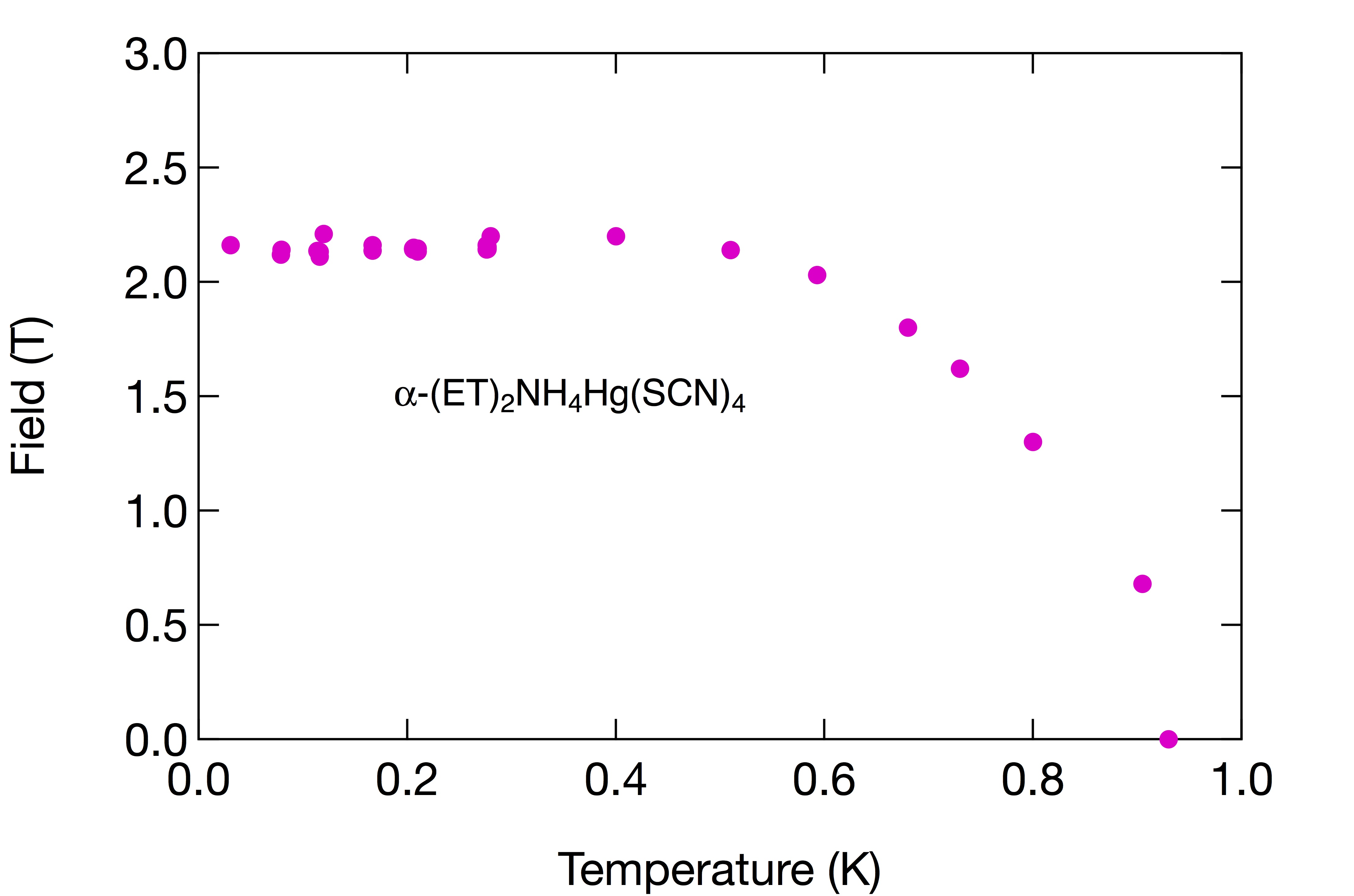$\alpha$-(ET)$_2$NH$_4$Hg(SCN)$_4$ This kappa phase material is a weak superconductor with just a 1 K $T_c$8. Rather than having an FFLO state it just reaches the Clogston-Chandrasakhar limit, also known as the Pauli paramagnetic limit ,$H_P$, and saturates at that field. The saturation is explained by the fact that the superconducting energy gap does not change much below $T_c/2$, so neither should the critical field if the superconductivity is being destroyed due to the Zeeman energy exceeding the superconducting gap energy.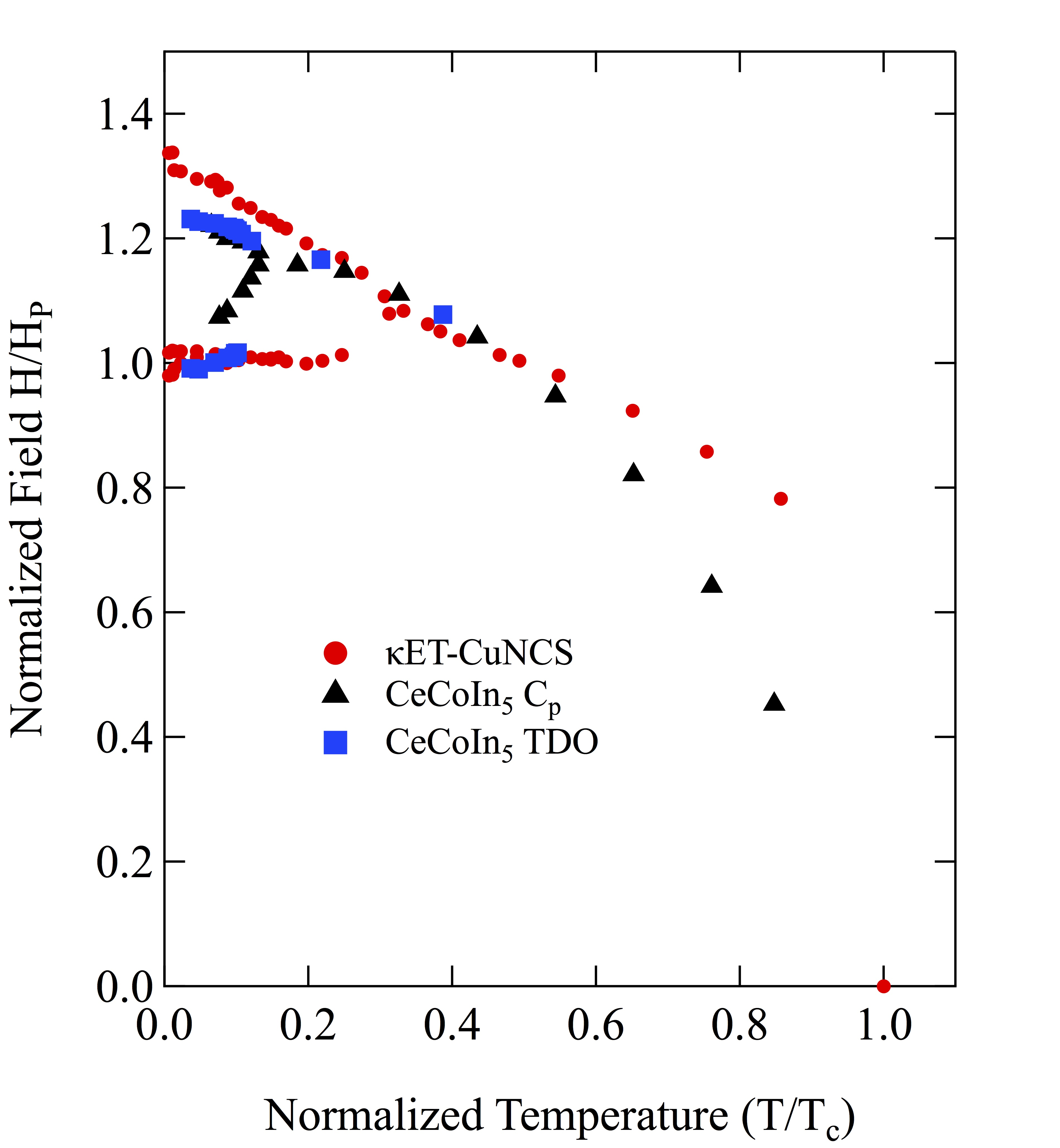CeCoIn$_5$ was one of the first materials where measurements suggested that there was a phase transition to inhomogeneous superconductivity9, 10. However subsequent measurements showed that the situation was more complicated, and although an FFLO state may exist in this heavy fermion material, it probably coexists with a spin density wave or some other correlated electron state. 11There is now a claim of an FFLO state in a the pnictide superconductor BaFeAs$_2$12. It has not been confirmed, and does not have the flat, zero slope, lower phase line (vortex state to FFLO state) that is predicted and measured in other materials. Further measurements are needed. Here it is plotted with data from $\kappa$-(BEDT-TTF)$_2$Cu(NCS)$_2$ normalized by $T_c$ and $H_P$.
As mentioned above the evidence is strongest in the crystalline organics. They are the most two dimensional and have the longest mean free paths of the materials that have been studied so far. More measurements in the pnictides may yield better results. It may help to intercalate some of the pnictides to make them more two dimensional, although that may reduce the mean free path. The optimal balance between lower dimensionality and mean free path remains a open question. There are many other systems that could be studied such as intercalated graphite, NbSe$_2$ and its variants, and possibly the cuprates. One would think the cuprates would be obvious candidates given their very high anisotropy, but for many of them the energy gap is prohibitively high to reach the magnetic fields necessary to reach the FFLO state.

All of the graphs above are normalized by the paramagnetic limit $H_P$. Also referred to as the Clogston Chandrasakhar limit a method of determining this value is found on this page.

_______________________________________________

1 Agosta, C.C. Jin, J. Coniglio, W.A. Smith, B.E. Cho, K. Stroe, I. Martin, C. Tozer, S.W. Murphy, T.P. Palm, E.C., "Experimental and semiempirical method to determine the Pauli-limiting field in quasi-two- dimensional superconductors as applied to κ-(BEDT-TTF)$_2$Cu(NCS)$_2$: Strong evidence of a FFLO state," Phys. Rev. B 85, 214514 (2012).
2 Lortz, R. Wang, Y. Demuer, A. Böttger, P.H.M. Bergk, B. Zwicknagl, G. Nakazawa, Y. Wosnitza, J. "Calorimetric Evidence for a Fulde-Ferrell-Larkin-Ovchinnikov Superconducting State in the Layered Organic Superconductor $\kappa$-(BEDT-TTF)$_2$Cu(NCS)$_2$," Phys. Rev. Lett. 99, 187002 (2007).
3 Agosta, C.C. Fortune, N.A. Hannahs, S.T. Gu, S. Liang, L. Park, J.H. Schleuter, J.A. "Calorimetric Measurements of Magnetic-Field-Induced Inhomogeneous Superconductivity Above the Paramagnetic Limit," Phys. Rev. Lett. 118, 267001 (2017).
4 Wright, J.A. Green, E. Kuhns, P. Reyes, A. Brooks, J. Schlueter, J. Kato, R. Yamamoto, H. Kobayashi, M. Brown, S.E., "Zeeman-Driven Phase Transition within the Superconducting State of $\kappa$-(BEDT-TTF)$_2$Cu(NCS)$_2$," Phys. Rev. Lett. 107, 087002 (2011).
5 Mayaffre, H. Kramer, S. Horvatic ́, M. Berthier, C. Miyagawa, K. Kanoda, K. Mitrovic ́, V.F. "Evidence of Andreev bound states as a hallmark of the FFLO phase in $\kappa$-(BEDT-TTF)$_2$Cu(NCS)$_2$," Nat. Phys. 10, 928 (2014).
6 Coniglio, W.A. Winter, L.E. Cho, K. Agosta, C.C. Fravel, B. Montgomery, L.K. Superconducting phase diagram and FFLO signature in $\lambda$-(BETS)$_2$GaCl$_4$ from rf penetration depth measurements. Phys. Rev. B 83, 224507 (2011).
7 Cho, K. Smith, B.E. Coniglio, W.A. Winter, L.E. Agosta, C.C. Schlueter, J.A. "Upper critical field in the organic superconductor $\beta$"-(BEDT-TTF)$_2$SF$_5$CH$_2$CF$_2$SO$_3$: Possibility of Fulde-Ferrell-Larkin-Ovchinnikov state," Phys. Rev. B 79, 220507 (2009).
8 Coffey, T. Martin, C. Agosta, C.C. Kinoshota, T. Tokumoto, M. "Bulk two-dimensional Pauli-limited superconductor," Phys. Rev. B 82, 212502 (2010).
9 Radovan, H.A., Fortune, N.A., Murphy, T.P. Hannahs, S.T. Palm, E.C., Tozer, S.W., Hall, D. "Magnetic enhancement of superconductivity from electron spin domains," Nature 425, 51 (2003). 10 Bianchi, A.; Movshovich, R.; Capan, C.; Pagliuso, P.G.; Sarrao, J.L. "Possible Fulde-Ferrell-Larkin-Ovchinnikov Superconducting State in CeCoIn5," Phys. Rev. Lett. 91, 187004 (2003). 11 Koutroulakis, G. Stewart, M.D.J. Mitrovic, V.F. Horvatic, M. Berthier, C. Lapertot, G. Flouquet, J. "Field Evolution of Coexisting Superconducting and Magnetic Orders in CeCoIn$_5$," Phys. Rev. Lett. 104, 087001 (2010).
12 Cho, C.W. Yang, J.H. Yuan, N.F.Q. Shen, J. Wolf, T. Lortz, R. "Thermodynamic Evidence for the Fulde-Ferrell-Larkin-Ovchinnikov State in the KFe$_2$As$_2$ Superconductor," Phys. Rev. Lett. 119, 593 (2017).
Last updated 11 Dec 2018
Webmaster
cagosta@clarku.edu